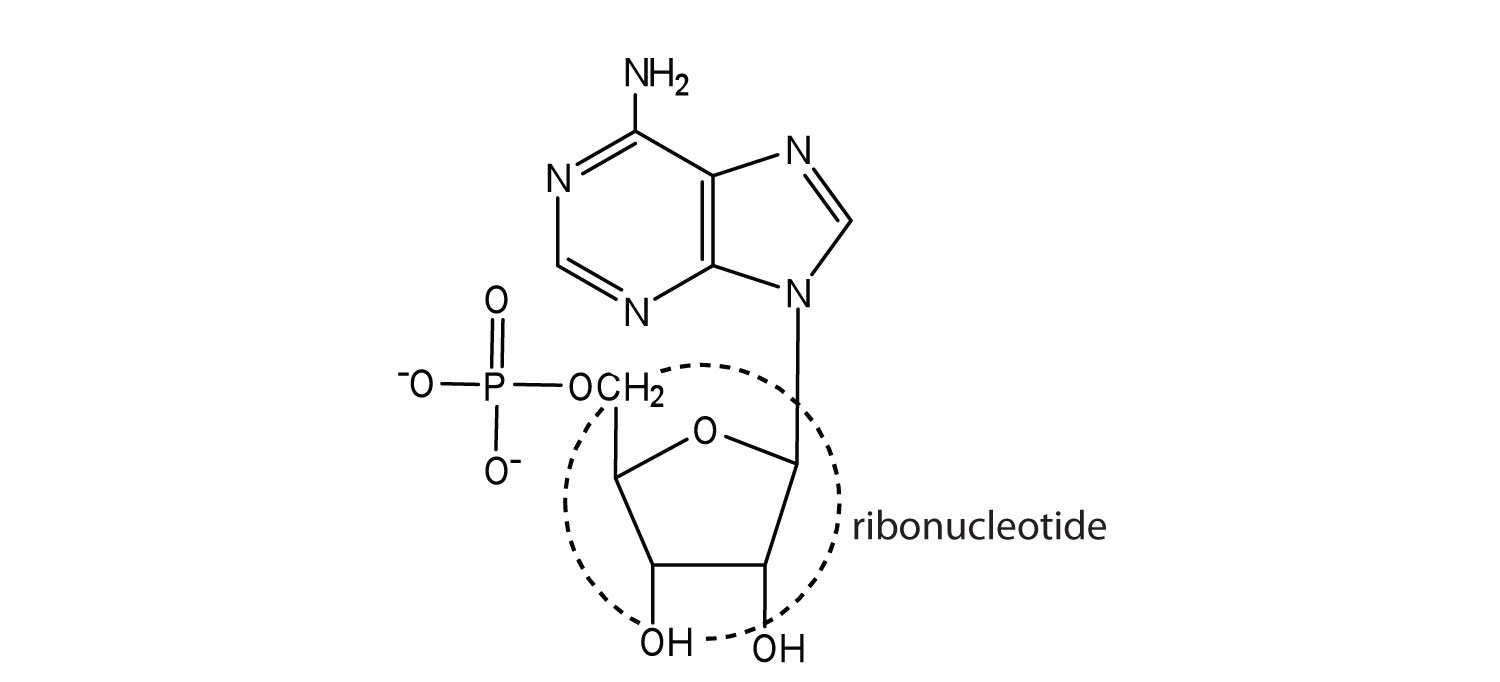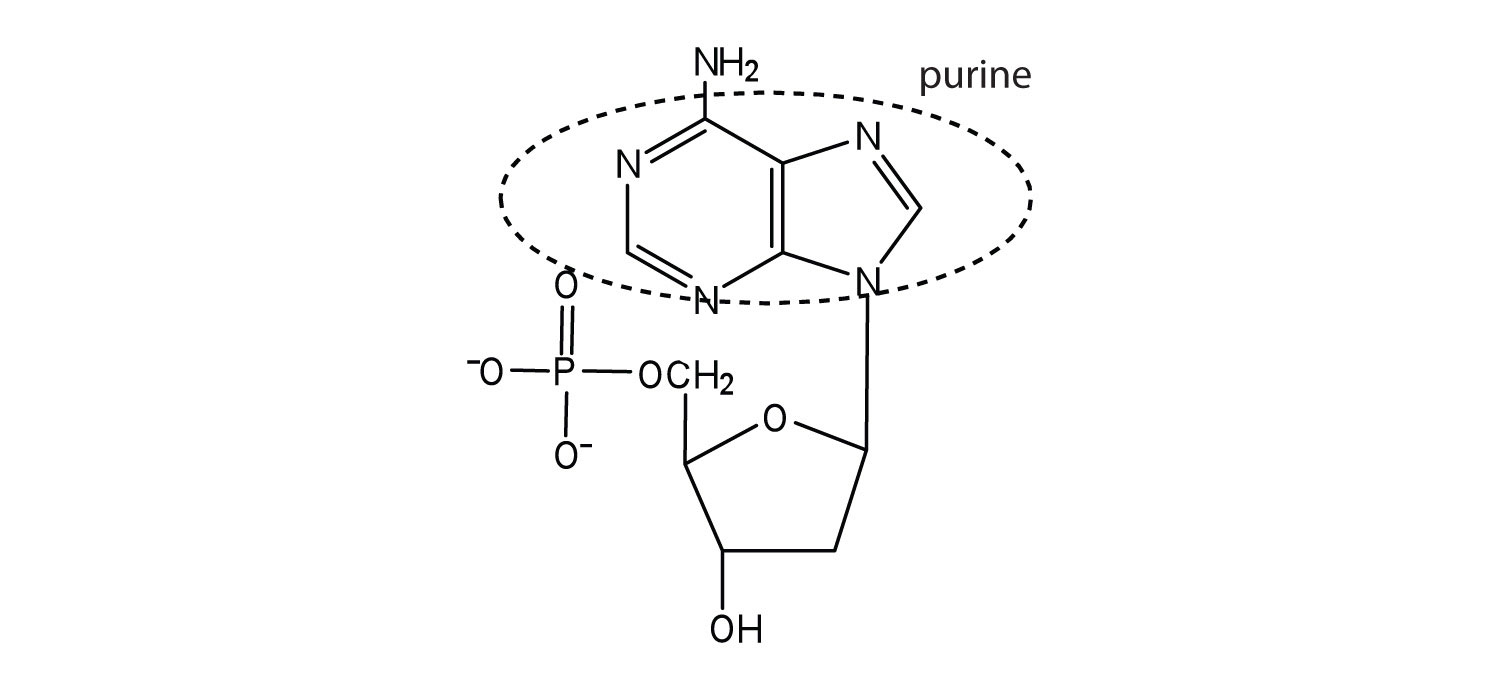# 5.2: Nucleotides

$$\newcommand{\vecs}{\overset { \rightharpoonup} {\mathbf{#1}} }$$ $$\newcommand{\vecd}{\overset{-\!-\!\rightharpoonup}{\vphantom{a}\smash {#1}}}$$$$\newcommand{\id}{\mathrm{id}}$$ $$\newcommand{\Span}{\mathrm{span}}$$ $$\newcommand{\kernel}{\mathrm{null}\,}$$ $$\newcommand{\range}{\mathrm{range}\,}$$ $$\newcommand{\RealPart}{\mathrm{Re}}$$ $$\newcommand{\ImaginaryPart}{\mathrm{Im}}$$ $$\newcommand{\Argument}{\mathrm{Arg}}$$ $$\newcommand{\norm}{\| #1 \|}$$ $$\newcommand{\inner}{\langle #1, #2 \rangle}$$ $$\newcommand{\Span}{\mathrm{span}}$$ $$\newcommand{\id}{\mathrm{id}}$$ $$\newcommand{\Span}{\mathrm{span}}$$ $$\newcommand{\kernel}{\mathrm{null}\,}$$ $$\newcommand{\range}{\mathrm{range}\,}$$ $$\newcommand{\RealPart}{\mathrm{Re}}$$ $$\newcommand{\ImaginaryPart}{\mathrm{Im}}$$ $$\newcommand{\Argument}{\mathrm{Arg}}$$ $$\newcommand{\norm}{\| #1 \|}$$ $$\newcommand{\inner}{\langle #1, #2 \rangle}$$ $$\newcommand{\Span}{\mathrm{span}}$$$$\newcommand{\AA}{\unicode[.8,0]{x212B}}$$

Learning Objectives

• To identify the different molecules that combine to form nucleotides.

The repeating, or monomer, units that are linked together to form nucleic acids are known as nucleotides. The deoxyribonucleic acid (DNA) of a typical mammalian cell contains about 3 × 109 nucleotides. Nucleotides can be further broken down to phosphoric acid (H3PO4), a pentose sugar (a sugar with five carbon atoms), and a nitrogenous base (a base containing nitrogen atoms).

$\mathrm{nucleic\: acids \underset{down\: into}{\xrightarrow{can\: be\: broken}} nucleotides \underset{down\: into}{\xrightarrow{can\: be\: broken}} H_3PO_4 + nitrogen\: base + pentose\: sugar}$

If the pentose sugar is ribose, the nucleotide is more specifically referred to as a ribonucleotide, and the resulting nucleic acid is ribonucleic acid (RNA). If the sugar is 2-deoxyribose, the nucleotide is a deoxyribonucleotide, and the nucleic acid is DNA.The nitrogenous bases found in nucleotides are classified as pyrimidines or purines. Pyrimidines are heterocyclic amines with two nitrogen atoms in a six-member ring and include uracil, thymine, and cytosine. Purines are heterocyclic amines consisting of a pyrimidine ring fused to a five-member ring with two nitrogen atoms. Adenine and guanine are the major purines found in nucleic acids (Figure $$\PageIndex{1}$$).Figure $$\PageIndex{1}$$ The Nitrogenous Bases Found in DNA and RNA

The formation of a bond between C1′ of the pentose sugar and N1 of the pyrimidine base or N9 of the purine base joins the pentose sugar to the nitrogenous base. In the formation of this bond, a molecule of water is removed. Table $$\PageIndex{1}$$ summarizes the similarities and differences in the composition of nucleotides in DNA and RNA.

The numbering convention is that primed numbers designate the atoms of the pentose ring, and unprimed numbers designate the atoms of the purine or pyrimidine ring.

Table $$\PageIndex{1}$$: Composition of Nucleotides in DNA and RNA
Composition DNA RNA
pyrimidine bases cytosine and thymine cytosine and uracil
pentose sugar 2-deoxyribose ribose
inorganic acid phosphoric acid (H3PO4) H3PO4

The names and structures of the major ribonucleotides and one of the deoxyribonucleotides are given in Figure $$\PageIndex{2}$$.Figure $$\PageIndex{2}$$: The Pyrimidine and Purine Nucleotides

Apart from being the monomer units of DNA and RNA, the nucleotides and some of their derivatives have other functions as well. Adenosine diphosphate (ADP) and adenosine triphosphate (ATP), shown in Figure $$\PageIndex{3}$$, have a role in cell metabolism. Moreover, a number of coenzymes, including flavin adenine dinucleotide (FAD), nicotinamide adenine dinucleotide (NAD+), and coenzyme A, contain adenine nucleotides as structural components.Figure $$\PageIndex{3}$$: Structures of Two Important Adenine-Containing Nucleotides

## Summary

Nucleotides are composed of phosphoric acid, a pentose sugar (ribose or deoxyribose), and a nitrogen-containing base (adenine, cytosine, guanine, thymine, or uracil). Ribonucleotides contain ribose, while deoxyribonucleotides contain deoxyribose.

## Concept Review Exercises

1. Identify the three molecules needed to form the nucleotides in each nucleic acid.

1. DNA
2. RNA
2. Classify each compound as a pentose sugar, a purine, or a pyrimidine.

2. guanine
3. deoxyribose
4. thymine
5. ribose
6. cytosine

1. nitrogenous base (adenine, guanine, cytosine, and thymine), 2-deoxyribose, and H3PO4
2. nitrogenous base (adenine, guanine, cytosine, and uracil), ribose, and H3PO4
1. purine
2. purine
3. pentose sugar
4. pyrimidine
5. pentose sugar
6. pyrimidine

## Exercises

1. What is the sugar unit in each nucleic acid?

1. RNA
2. DNA
2. Identify the major nitrogenous bases in each nucleic acid.

1. DNA
2. RNA
3. For each structure, circle the sugar unit and identify the nucleotide as a ribonucleotide or a deoxyribonucleotide.

1.2.4. For each structure, circle the sugar unit and identify the nucleotide as a ribonucleotide or a deoxyribonucleotide.

1.2.5. For each structure, circle the nitrogenous base and identify it as a purine or pyrimidine.

1.2.6. For each structure, circle the nitrogenous base and identify it as a purine or pyrimidine.

1.2.1.2.1.2.